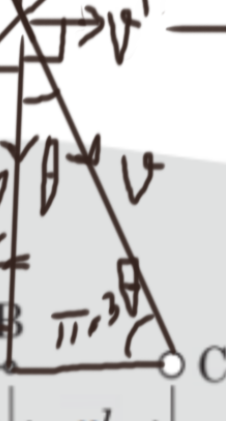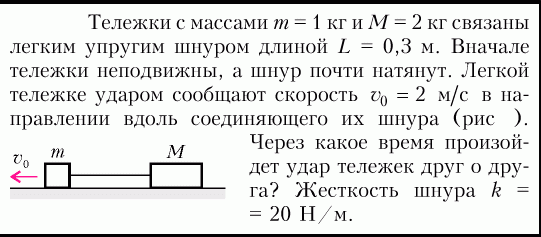This is a question from an olympiad workbook. Where can I find more such questions involving almost inextensible elastic cords? Answer: 30 degrees

1 симпатия

The main idea of solving the problem is that the energy is conserved and the speed will remain the same, for the time being, the length of the cord will also remain the same. Because of this, we can use geometry. We will have several triangles:

Then you can do different things, for example, take this triangleand use the sine theorem.
From the ratio, an equation will be obtained from which it will be possible to get an answer of 30 degrees.

4 симпатии

Thanks a lot! Do you know about any source where I can practice more problems of almost stretched elastic cords like these problems?Once I was advised to use the PhysOlymp. I think it is quite useful in preparing for exams and Olympiads, but personally, I have never used it. It’s in English.
You can also use Khanacademy and Mathus websites, but Mathus is in Russian. There are a lot of different handouts on various topics, including this one (1, 2)

1 симпатия

Thank you!

1 симпатия